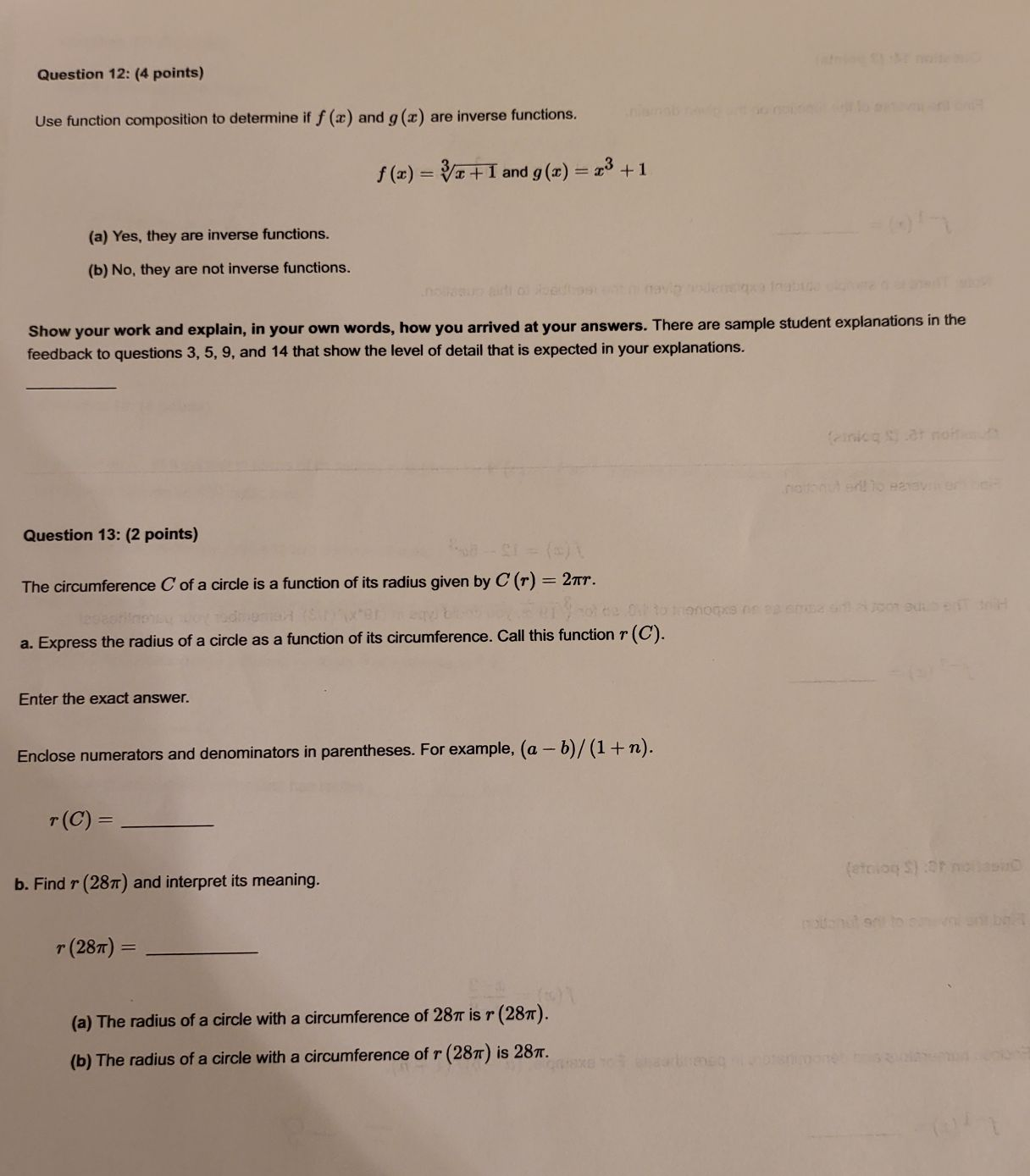### ¿Todavía tienes preguntas de matemáticas?

Pregunte a nuestros tutores expertos
Algebra
PreguntaUse function composition to determine if $$f ( x )$$ and $$g ( x )$$ are inverse functions. $$f ( x ) = \sqrt[ 3 ] { x + 1 }$$ and $$g ( x ) = x ^ { 3 } + 1$$ (a) Yes, they are inverse functions. (b) No, they are not inverse functions. Show your work and explain, in your own words, how you arrived at your answers. There are sample student explanations in the feedback to questions $$3,5,9$$ , and $$14$$ that show the level of detail that is expected in your explanations.